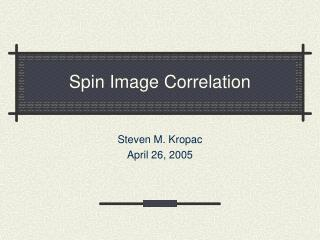DownloadDownload PresentationSpin Image Correlation

# Spin Image Correlation

Télécharger la présentation## Spin Image Correlation

- - - - - - - - - - - - - - - - - - - - - - - - - - - E N D - - - - - - - - - - - - - - - - - - - - - - - - - - -
##### Presentation Transcript

1. Spin Image Correlation Steven M. Kropac April 26, 2005

2. Presentation contents • Overview • Background/Motivation • Operational Concept • Functional Diagram • Software Implementation • Application • Conclusion

3. Overview • A spin image provides a 2D object centered description of a 3D mesh object. • Essentially, a spin image represents the radial and elevation distances to every other vertex on the mesh. • Resultant spin images can be compared (correlated) to identify similarities between vertices.

4. Background/Motivation • Object recognition for 3D computer vision has many applications in the area of robotics and automation. • Andrew Johnson works on Surface System Guidance Navigation and Controlfor the mars science laboratory (2009) • “Using Spin Images for Efficient Object Recognition in Cluttered 3D Scenes” –Andrew E. Johnson and Martial Hebert (5/1999) • Presents an approach for recognition of complex objects in cluttered 3-D scenes. • Encoding relative positions of many points on the surface is not new. Others have performed explored using view-based approaches, or storing points along curves. • The approach described in this presentation is different because the spin images are much more discriminating than curves and angles and is object centered, so it is not view dependant.

5. Operational Concept • Provide a Graphical User Interface (GUI) for loading 3D mesh models. • Models are specified in .ply file format • Two operations available to user: • Generate spin images for every vertex on a loaded 3D mesh model • Perform spin image correlation between a selected vertex and every other vertex on the model. • Highly correlated vertexes will be colored bright green • Vertices with limited correlation will be colored very dark green or black (background color).

6. Functional DiagramGenerate Spin images

7. Spin Image calculations • Start with a selected vertex and associated normal, which we call an oriented point. • Calculate alpha, beta distances from the oriented point to every other vertex. • Alpha = perpendicular distance from surface normal • Beta = signed perpendicular distance to the plane p

8. Spin Image calculationsCalculating alpha,beta p – oriented point n – surface normal x – position of another vertex on mesh Beta – difference of x - p, then dot product with surface normal to project on Normal. Alpha – length of vector from x to p, subtract out Beta component Distance between points = sum of square of differences

9. Spin Image calculationssample alpha, beta values

10. Spin Image calculationsGenerate spin images • A bin size (i,j) is selected as a multiple of the resolution of the mesh. • My application uses 1x. • The alpha,beta values are then translated to the appropriate bin. • Bin dimensions might be 4x20. Alpha Beta ranges might be [0,2] and [-3,3]. • When an alpha,beta pair is translated the associated bin is incremented.

11. Spin Image calculationssample spin image bins

12. Functional DiagramPerform Spin-image Correlation

13. Spin Image CorrelationCalculating correlation coefficient • Correlation coefficient provides the quality of a least squares fitting to the selected data set.

14. Software Implementatoin • The spin image application is implemented in C++ using Microsoft Visual Studio. • The display of 3D mesh objects is accomplished using OpenGL. • A MySQL database is utilized to store calculation of spin images. • Database stores (filename, vertexIndex, alphaBucket, betaBucket, intensityValue) • For an image with 5000 vertices and a spin image size of 85x42 represents ~1.8 million database entries. • Spin Image calculations takes several minutes The amount of time is dependant on the number of vertices in the model.

15. Spin Image Application • The application provides the capability to parse .ply graphics files and display the 3D model using OpenGL.

16. Spin Image Applicationkeyboard commands Left/right arrow – rotate along y axis Up/down arrow – rotate along x axis PgUp/PgDn – rotate along z axis -/= zoom-out / zoom-in Home/end – select next/previous vertex

17. Spin Image ApplicationPull-down menu features • File • Open .ply file • Exit • Operation • Calculate Spin Images and store in Database • Display Spin Image Correlations • View • Zoom-in • Zoom-out

18. Spin Image ApplicationResults Selected vertex

19. Spin Image ApplicationResults (elephant) • 5+ hours to generate spin images • 10 minutes to spin-correlate Selected vertex

20. Conclusion • Spin images provides an interesting representation for surface matching. • More work needs to be done to fully understand how speed issues can be addressed. • Ex: A 3D mesh has 5000 vertices. To calculate all spin images for this model requires 5000 * 5000 = 25 million sets of calculations.